# Real-time dynamical system

## Definition

A real-time dynamical system or a real dynamical system or flow is a triple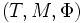$(T,M,\Phi)$ where:

•$T$ is an open nonempty interval in the reals, containing zero
•$M$ is a differential manifold
•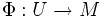$\Phi:U \to M$ is a map where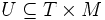$U \subseteq T \times M$

such that: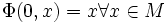$\Phi(0,x) = x \forall x \in M$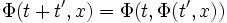$\Phi(t + t',x) = \Phi(t,\Phi(t',x))$ if both sides are defined

### Terminology

To each$x$ let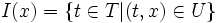$I(x) = \{ t \in T| (t,x) \in U \}$. Then$I(x)$ is open in$T$. We can them define a map: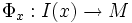$\Phi_x: I(x) \to M$ given as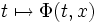$t \mapsto \Phi(t,x)$.

•$\Phi$ is termed the evolution function
•$M$ is called the phase space or state space
•$t$ is called the evolution parameter
•$x$ is called the initial state of the system
• The map$\Phi_x$ is termed the flow at$x$ and its graph the trajectory through$x$. Its image is termed the orbit of$x$
• A subspace$S$ of$M$ is said to be$\Phi$-invariant if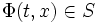$\Phi(t,x) \in S$ for all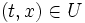$(t,x) \in U$ for which$x \in S$.